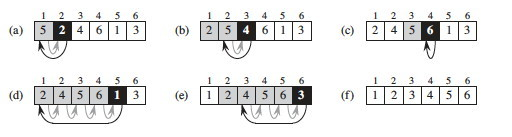## Algorithms in Go: Insertion Sort

Posted on December 29, 2019 | 2 minute read

### Introduction

One of the most basic sorting algorithms is the insertion sort. It is also relatively easy to understand as it closely mimics a common sorting strategy that humans normally use when sorting playing cards in their hand. Even though its time complexity is O(n2) for the worst case, it still performs well for small input sizes. Below is an illustration of the steps taken to perform a merge sort:### Implementation

The main two parts of the code below are the two `for` loops. In the first one, we go through every element of the initial slice, starting with the second (see (a) above) and we compare it iteratively backwards with the previous elements. Whenever the previous element is greater than the current one, we move it one position forward. Once the inner loop reaches the condition to stop, we assign the value of the current element to precede the last element that was moved forward. By doing this, we end up with a sorted slice.

``````func Sort(a []int) []int {
// creating a new slice of a size equal to the original
result := make([]int, len(a))
// copying elements from the original slice to the new slice
copy(result, a)
// iterating through all elements of the slice starting with the second
for i := 1; i < len(result); i++ {
// key holds the current value
key := result[i]
// j holds the index of the previous value
j := i - 1
// we iterate backwards starting with the previous value and compare it with the current value
for j >= 0 && result[j] > key {
// if the previous value is greater then we move it forward one position
result[j+1] = result[j]
// we decrement the index for the previous value so that we check the next value backwards
j--
}
// at the end of the inner loop we want to potentially move the current value of the main loop to a new position (if anything was shifted above)
result[j+1] = key
}
// returning the sorted slice
return result
}
``````

### Go specific decisions

As Go does not make a copy of the slice after it has been passed into a function, we had to make sure that we are no mutating the underlying array of the original slice and therefore had to create a new slice of the size of the original and copy into it the numbers.

### Source code

https://github.com/dorin131/go-algorithms

Please feel free to make PRs with improvements.

More algorithms are coming soon!

```tags:go algorithms sort insertion sort ```